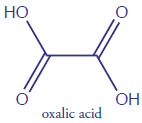# 2.3: Stoichiometric Calculations

•• Contributed by David Harvey
• Professor (Chemistry and Biochemistry) at DePauw University

A balanced reaction, which gives the stoichiometric relationship between the moles of reactants and the moles of products, provides the basis for many analytical calculations. Consider, for example, an analysis for oxalic acid, $$\ce{H_2C_2O_4}$$, in which $$\ce{Fe^{3+}}$$ oxidizes oxalic acid to $$\ce{CO_2}$$.

$\ce{2Fe^{3+}}(aq) + \ce{H2C2O4}(aq) + \ce{2H2O}(l) \rightarrow \ce{2Fe^2+}(aq) + \ce{2CO2}(g) + \ce{2H3O+}(aq)$

The balanced reaction indicates that one mole of oxalic acid reacts with two moles of $$\ce{Fe^{3+}}$$. As shown in Example $$\PageIndex{1}$$, we can use this balanced reaction to determine the amount of oxalic acid in a sample of rhubarb.

Example $$\PageIndex{1}$$

The amount of oxalic acid in a sample of rhubarb was determined by reacting with $$\ce{Fe^{3+}}$$. After extracting a 10.62 g of rhubarb with a solvent, oxidation of the oxalic acid required 36.44 mL of 0.0130 M $$\ce{Fe^{3+}}$$. What is the weight percent of oxalic acid in the sample of rhubarb?Figure $$\PageIndex{1}$$: Oxalic acid

SOLUTION

We begin by calculating the moles of $$\ce{Fe^{3+}}$$ used in the reaction

$\mathrm{\dfrac{0.0130\: mol\: Fe^{3+}}{L} \times 0.03644\: L = 4.73{\color{Red} 7} \times 10^{-4}\: mol\: Fe^{3+}}$

The moles of oxalic acid reacting with the $$\ce{Fe^{3+}}$$, therefore, is

$\mathrm{4.73{\color{Red} 7}\times10^{-4}\: mol\: Fe^{3+} \times \dfrac{1\: mol\: H_2C_2O_4}{2\: mol\: Fe^{3+}} = 2.36{\color{Red} 8} \times 10^{-4}\: mol\: H_2C_2O_4}$

Converting the moles of oxalic acid to grams of oxalic acid

$\mathrm{2.36{\color{Red} 8}\times10^{-4}\: mol\: C_2H_2O_4 \times \dfrac{90.03\: g\: H_2C_2O_4}{mol\: H_2C_2O_4} = 2.13{\color{Red} 2}\times10^{-2}\: g\: H_2C_2O_4}$

and calculating the weight percent gives the concentration of oxalic acid in the sample of rhubarb as

$\mathrm{\dfrac{2.13{\color{Red} 2}\times10^{-2}\: g\: H_2C_2O_4}{10.62\: g\: rhubarb}\times100 = 0.201\%\: \textrm{w/w}\: H_2C_2O_4}$

Note: Oxalic acid, in sufficient amounts, is toxic. At lower physiological concentrations it leads to the formation of kidney stones. The leaves of the rhubarb plant contain relatively high concentrations of oxalic acid. The stalk, which many individuals enjoy eating, contains much smaller concentrations of oxalic acid.

Note

Note that we retain an extra significant figure throughout the calculation, rounding to the correct number of significant figures at the end. We will follow this convention in any problem involving more than one step. If we forget that we are retaining an extra significant figure, we might report the final answer with one too many significant figures. In this chapter we will mark the extra digit in red for emphasis. Be sure that you pick a system for keeping track of significant figures.

The analyte in Example $$\PageIndex{1}$$, oxalic acid, is in a chemically useful form because there is a reagent, $$\ce{Fe^{3+}}$$, that reacts with it quantitatively. In many analytical methods, we must convert the analyte into a more accessible form before we can complete the analysis. For example, one method for the quantitative analysis of disulfiram, $$\ce{C_{10}H_{20}N_2S_4}$$—the active ingredient in the drug Anatbuse—requires that we convert the sulfur to $$\ce{H_2SO_4}$$ by first oxidizing it to $$\ce{SO_2}$$ by combustion, and then oxidizing the $$\ce{SO_2}$$ to $$\ce{H_2SO_4}$$ by bubbling it through a solution of $$\ce{H_2O_2}$$. When the conversion is complete, the amount of $$\ce{H_2SO_4}$$ is determined by titrating with $$\ce{NaOH}$$.Figure $$\PageIndex{2}$$: Disulfiram

To convert the moles of $$\ce{NaOH}$$ used in the titration to the moles of disulfiram in the sample, we need to know the stoichiometry of the reactions. Writing a balanced reaction for $$\ce{H_2SO_4}$$ and $$\ce{NaOH}$$ is straightforward

$\ce{H2SO4}(aq) + \ce{2NaOH}(aq) \rightarrow \ce{2H2O}(l) + \ce{Na2SO4}(aq)$

but the balanced reactions for the oxidations of $$\ce{C_{10}H_{20}N_2S_4}$$ to $$\ce{SO_2}$$, and of $$\ce{SO_2}$$ to $$\ce{H_2SO_4}$$ are not as immediately obvious. Although we can balance these redox reactions, it is often easier to deduce the overall stoichiometry by using a little chemical logic.

Exercise $$\PageIndex{1}$$

You can dissolve a precipitate of $$\ce{AgBr}$$ by reacting it with $$\ce{Na_2S_2O_3}$$, as shown here.

$\ce{AgBr}(s) + \ce{2Na2S2O3}(aq) \rightarrow \ce{Ag(S2O3)2^3-}(aq) + \ce{Br-}(aq) + \ce{4Na+}(aq)$

How many mL of 0.0138 M $$\ce{Na_2S_2O_3}$$ do you need to dissolve 0.250 g of $$\ce{AgBr}$$?

Example $$\PageIndex{2}$$

An analysis for disulfiram, $$\ce{C_{10}H_{20}N_2S_4}$$, in Antabuse is carried out by oxidizing the sulfur to $$\ce{H_2SO_4}$$ and titrating the $$\ce{H_2SO_4}$$ with $$\ce{NaOH}$$. If a 0.4613-g sample of Antabuse is taken through this procdure, requiring 34.85 mL of 0.02500 M $$\ce{NaOH}$$ to titrate the $$\ce{H_2SO_4}$$, what is the $$\%\: \textrm{w/w}$$ disulfiram in the sample?

SOLUTION

Calculating the moles of $$\ce{H_2SO_4}$$ is easy—first, we calculate the moles of $$\ce{NaOH}$$ used in the titration

$\mathrm{(0.02500\: M)×(0.03485\: L) = 8.712{\color{Red} 5}\times10^{-4}\: mol\: NaOH}$

and then we use the balanced reaction to calcualte the corresponding moles of $$\ce{H_2SO_4}$$.

$\mathrm{8.712{\color{Red} 5}\times10^{-4}\: mol\: NaOH \times \dfrac{1\:mol\: H_2SO_4}{2\: mol\: NaOH} = 4.356{\color{Red} 2}\times10^{-4}\: mol\: H_2SO_4}$

We do not need balanced reactions to convert the moles of $$\ce{H_2SO_}$$4 to the corresponding moles of $$\ce{C_{10}H_{20}N_2S_4}$$. Instead, we take advantage of a conservation of mass—all the sulfur in $$\ce{C_{10}H_{20}N_2S_4}$$ must end up in the H2SO4(Here is where we use a little chemical logic! A conservation of mass is the essence of stoichiometry.); thus

$\mathrm{4.356{\color{Red} 2}×10^{-4}\: mol\: \ce{H2SO4} \times \dfrac{1\:mol\: S}{mol\: \ce{H2SO4}} \times \dfrac{1\:mol\: \ce{C10H20N2S4}}{4\: mol\: S} = 1.089{\color{Red} 0}\times10^{-4}\: mol\: \ce{C10H20N2S4}}$

or

$\mathrm{1.089{\color{Red} 0}×10^{-4}\: mol\: \ce{C10H20N2S4} × \dfrac{296.54\: g\: \ce{C10H20N2S4}}{mol\: \ce{C10H20N2S4}} = 0.03229{\color{Red} 3}\: g\: \ce{C10H20N2S4}}$

$\mathrm{\dfrac{0.03229{\color{Red} 3}\: g\: \ce{C10H20N2S4}}{0.4613\: g\: sample} × 100 = 7.000\%\: \textrm{w/w}\: \ce{C10H20N2S4}}$